Next: Using vectors
Up: EXPLANATION AND EXAMPLES
Previous: Formatting angles

## Vectors and Matrices

As an alternative to employing a spherical polar coordinate system, the direction of an object can be defined in terms of the sum of any three vectors as long as they are different and not coplanar. In practice, three vectors at right angles are usually chosen, forming a system of Cartesian coordinates. The x- and y-axes lie in the fundamental plane (e.g. the equator in the case of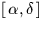), with the x-axis pointing to zero longitude. The z-axis is normal to the fundamental plane and points towards positive latitudes. The y-axis can lie in either of the two possible directions, depending on whether the coordinate system is right-handed or left-handed. The three axes are sometimes called a triad. For most applications involving arbitrarily distant objects such as stars, the vector which defines the direction concerned is constrained to have unit length. The x-, y- and z-components can be regarded as the scalar (dot) product of this vector onto the three axes of the triad in turn. Because the vector is a unit vector, each of the three dot-products is simply the cosine of the angle between the unit vector and the axis concerned, and the x-, y- and z-components are sometimes called direction cosines.

For some applications involving objects with the Solar System, unit vectors are inappropriate, and it is necessary to use vectors scaled in length-units such as AU, km etc. In these cases the origin of the coordinate system may not be the observer, but instead might be the Sun, the Solar-System barycentre, the centre of the Earth etc. But whatever the application, the final direction in which the observer sees the object can be expressed as direction cosines.

But where has this got us? Instead of two numbers - a longitude and a latitude - we now have three numbers to look after - the x-, y- and z-components - whose quadratic sum we have somehow to contrive to be unity. And, in addition to this apparent redundancy, most people find it harder to visualize problems in terms of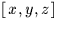than in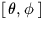.Despite these objections, the vector approach turns out to have significant advantages over the spherical trigonometry approach:

• Vector formulae tend to be much more succinct; one vector operation is the equivalent of strings of sines and cosines.
• The formulae are as a rule rigorous, even at the poles.
• Accuracy is maintained all over the celestial sphere. When one Cartesian component is nearly unity and therefore insensitive to direction, the others become small and therefore more precise.
• Formulations usually deliver the quadrant of the result without the need for any inspection (except within the library function ATAN2).
A number of important transformations in positional astronomy turn out to be nothing more than changes of coordinate system, something which is especially convenient if the vector approach is used. A direction with respect to one triad can be expressed relative to another triad simply by multiplying thecolumn vector by the appropriate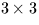orthogonal matrix (a tensor of Rank 2, or dyadic). The three rows of this rotation matrix are the vectors in the old coordinate system of the three new axes, and the transformation amounts to obtaining the dot-product of the direction-vector with each of the three new axes. Precession, nutation,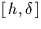to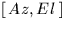,to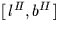and so on are typical examples of the technique. A useful property of the rotation matrices is that they can be inverted simply by taking the transpose.

The elements of these vectors and matrices are assorted combinations of the sines and cosines of the various angles involved (hour angle, declination and so on, depending on which transformation is being applied). If you write out the matrix multiplications in full you get expressions which are essentially the same as the equivalent spherical trigonometry formulae. Indeed, many of the standard formulae of spherical trigonometry are most easily derived by expressing the problem initially in terms of vectors.Next: Using vectors
Up: EXPLANATION AND EXAMPLES
Previous: Formatting angles

SLALIB --- Positional Astronomy Library